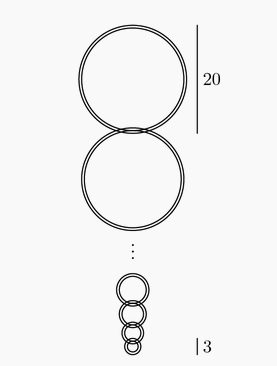###### back to index | new

A large candle is $119$ centimeters tall. It is designed to burn down more quickly when it is first lit and more slowly as it approaches its bottom. Specifically, the candle takes $10$ seconds to burn down the first centimeter from the top, $20$ seconds to burn down the second centimeter, and $10k$ seconds to burn down the $k$-th centimeter. Suppose it takes $T$ seconds for the candle to burn down completely. Then $\tfrac{T}{2}$ seconds after it is lit, the candle's height in centimeters will be $h$. Find $10h$.

The terms of an arithmetic sequence add to $715$. The first term of the sequence is increased by $1$, the second term is increased by $3$, the third term is increased by $5$, and in general, the $k$th term is increased by the $k$th odd positive integer. The terms of the new sequence add to $836$. Find the sum of the first, last, and middle terms of the original sequence.

Two geometric sequences $a_1, a_2, a_3, \ldots$ and $b_1, b_2, b_3, \ldots$ have the same common ratio, with $a_1 = 27$, $b_1=99$, and $a_{15}=b_{11}$. Find $a_9$.

The degree measures of the angles in a convex 18-sided polygon form an increasing arithmetic sequence with integer values. Find the degree measure of the smallest angle.

The sum of the first 2011 terms of a geometric sequence is 200. The sum of the first 4022 terms is 380. Find the sum of the first 6033 terms.

A bee starts flying from point $P_0$. She flies $1$ inch due east to point $P_1$. For $j \ge 1$, once the bee reaches point $P_j$, she turns $30^{\circ}$ counterclockwise and then flies $j+1$ inches straight to point $P_{j+1}$. When the bee reaches $P_{2015}$ she is exactly $a \sqrt{b} + c \sqrt{d}$ inches away from $P_0$, where $a$, $b$, $c$ and $d$ are positive integers and $b$ and $d$ are not divisible by the square of any prime. What is $a+b+c+d$ ?

Consider an arithmetic sequence with $a_3 = 165$ and $a_{12} = 615$. For what value of n is $a_n = 2015$?

The first three terms of a geometric progression are $\sqrt 3$, $\sqrt3$, and $\sqrt3$. What is the fourth term?

Let $a < b < c$ be three integers such that $a,b,c$ is an arithmetic progression and $a,c,b$ is a geometric progression. What is the smallest possible value of $c$?

The number $2017$ is prime. Let $S = \sum \limits_{k=0}^{62} \dbinom{2014}{k}$. What is the remainder when $S$ is divided by $2017$?

The sequence $\log_{12}{162}$, $\log_{12}{x}$, $\log_{12}{y}$, $\log_{12}{z}$, $\log_{12}{1250}$ is an arithmetic progression. What is $x$?

Jo and Blair take turns counting from $1$ to one more than the last number said by the other person. Jo starts by saying "$1$", so Blair follows by saying "$1, 2$". Jo then says "$1, 2, 3$", and so on. What is the $53^{\text{rd}}$ number said?

An arithmetic sequence has first term $a$ and common difference $d$. If the sum of the first ten terms is half the sum of the next ten terms, what is the ratio $\frac{a}{d}$ ? Express your answer as a common fraction.

In the eight term sequence $A$, $B$, $C$, $D$, $E$, $F$, $G$, $H$, the value of $C$ is $5$ and the sum of any three consecutive terms is $30$. What is $A+H$?

The arithmetic mean of two distinct positive integers $x$ and $y$ is a two-digit integer. The geometric mean of $x$ and $y$ is obtained by reversing the digits of the arithmetic mean. What is $|x - y|$?

The first four terms of an arithmetic sequence are $p$, $9$, $3p-q$, and $3p+q$. What is the $2010^\text{th}$ term of this sequence?

Arithmetic sequences $\left(a_n\right)$ and $\left(b_n\right)$ have integer terms with $a_1=b_1=1 < a_2 \le b_2$ and $a_n b_n = 2010$ for some $n$. What is the largest possible value of $n$?

A geometric sequence $(a_n)$ has $a_1=\sin x$, $a_2=\cos x$, and $a_3= \tan x$ for some real number $x$. For what value of $n$ does $a_n=1+\cos x$?

The first three terms of an arithmetic sequence are $2x - 3$, $5x - 11$, and $3x + 1$ respectively. The $n$th term of the sequence is $2009$. What is $n$?

Let $a + ar_1 + ar_1^2 + ar_1^3 + \cdots$ and $a + ar_2 + ar_2^2 + ar_2^3 + \cdots$ be two different infinite geometric series of positive numbers with the same first term. The sum of the first series is $r_1$, and the sum of the second series is $r_2$. What is $r_1 + r_2$?

The fifth and eighth terms of a geometric sequence of real numbers are $7!$ and $8!$ respectively. What is the first term?

Let $a, b, c, d$, and $e$ be five consecutive terms in an arithmetic sequence, and suppose that $a+b+c+d+e=30$. Which of $a, b, c, d,$ or $e$ can be found?

The geometric series $a+ar+ar^2\ldots$ has a sum of $7$, and the terms involving odd powers of $r$ have a sum of $3$. What is $a+r$?

A number of linked rings, each 1 cm thick, are hanging on a peg. The top ring has an outside diameter of 20 cm. The outside diameter of each of the outer rings is 1 cm less than that of the ring above it. The bottom ring has an outside diameter of 3 cm. What is the distance, in cm, from the top of the top ring to the bottom of the bottom ring?Big Al, the ape, ate 100 bananas from May 1 through May 5. Each day he ate six more bananas than on the previous day. How many bananas did Big Al eat on May 5?